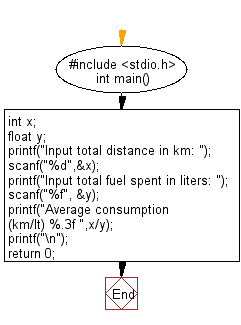﻿ C : Average consumption from total distance and fuel spent

# C Exercises: Calculate average consumption from the given total distance and fuel spent

## C Basic Declarations and Expressions: Exercise-14 with Solution

Write a C program to calculate a bike’s average consumption from the given total distance (integer value) travelled (in km) and spent fuel (in litters, float number – 2 decimal points).

C Code:

``````#include <stdio.h>
int main()
{
int x;    // Variable to store total distance in km
float y;  // Variable to store total fuel spent in liters

// Prompt user for total distance and store in 'x'
printf("Input total distance in km: ");
scanf("%d",&x);

// Prompt user for total fuel spent and store in 'y'
printf("Input total fuel spent in liters: ");
scanf("%f", &y);

// Calculate and print average consumption
printf("Average consumption (km/lt) %.3f ",x/y);
printf("\n");

return 0;
}
``````

Sample Output:

```Input total distance in km: 350
Input total fuel spent in liters: 5
Average consumption (km/lt) 70.000
```

Flowchart:C Programming Code Editor:

What is the difficulty level of this exercise?

Test your Programming skills with w3resource's quiz.

﻿